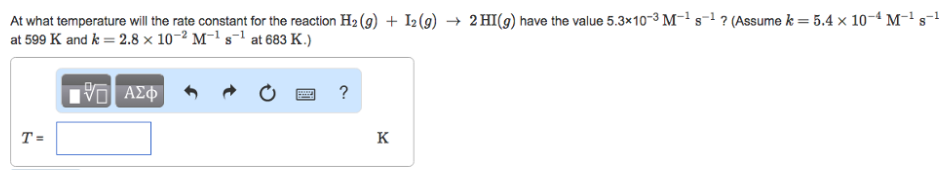# Problem: At what temperature will the rate constant for the reaction H2 (g) + I2 (g) --&gt;HI (g)  have the value 5.3x10-3 M-1 s-1? (Assume k = 5.4x10-4 M-1 s-1 at 599 K and k = 2.8x10-2 M-1 s-1 at 683 K.)

###### FREE Expert Solution
81% (28 ratings)###### Problem Details

At what temperature will the rate constant for the reaction H2 (g) + I2 (g) -->HI (g)  have the value 5.3x10-3 M-1 s-1? (Assume k = 5.4x10-4 M-1 s-1 at 599 K and k = 2.8x10-2 M-1 s-1 at 683 K.)